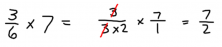Smartick is an advanced online program that teaches kids math and coding in only 15 min. a day

Apr14

# Learn and Practice How to Multiply Fractions

In this post, we are going to learn how to multiply fractions (two or more). To do this, we only have to follow these steps:

1. Simplify fractions: Any numerator can be simplified with any denominator.
2. Multiply fractions in a line: Multiply the denominators to obtain the final denominator and multiply the numerators to obtain the final numerator.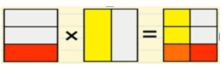For example: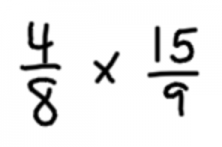First, we should simplify the fractions so that they are easier to multiply afterward.  So to simplify, we will factor each number into its prime factors.

4 = 2 x 2

8 = 2 x 2 x 2

15 = 3 x 5

9 = 3 x 3

And we replace each number in the fractions with its prime factors.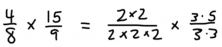Now we simplify, crossing out the numerators and denominators that are the same. We are left with the result of the multiplication which is 5/6.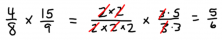### How to perform a multiplication of fractions with a whole number:

Multiplying a fraction by a whole number is very simple, we make the whole number into a fraction by putting 1 in the place of the denominator.

For example: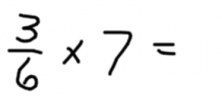The fraction 3/6 can be simplified as we have seen in the previous example by breaking it down into its prime factors and we are left with 1/2.

We make the whole number 7 into a fraction by using 1 as the denominator: 7/1.

Now we multiply in a line: we multiply the denominators: 2 x 1 = 2.

We multiply the numerators: 1 x 7 = 7

In this way, we are left with the fraction: 7 / 2